Technical Report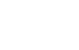Vibration and Sensors II

(5) LV series of Laser Doppler Vibrometers - Velocity Sensors -

A laser Doppler vibrometer consists of an optical head that emits laser light and a converter that processes the Doppler frequency of the reflected laser light. The voltage signal from the converter is proportional to the velocity at which the object under measurement moves. This signal is input to an FFT analyzer or an alternative type of instrument to convert the signal into an acceleration or displacement signal. In addition, frequency analysis can be performed on the signal to examine and gain a better understanding of how the object behaves.

Principle of Operation

If an acoustic, radio or light wave of a specific frequency is beamed at a moving object, the frequency of the wave reflected from the moving object differs in proportion to the velocity of the object. This phenomenon is known as Doppler shift or the Doppler effect. The relationship between the frequency at which the wave is beamed and the frequency at which the wave echoes is outlined below.

1. If the object is approaching the source, the reflected frequency is higher than the emitted frequency.

2. If the object is receding from the source, the reflected frequency is lower than the emitted frequency.

The difference between the eitted frequency and the reflected frequency relates to the velocity of the moving object. Normally, the difference in frequency becomes larger as the velocity of the object increases.

The laser Doppler vibrometer is based on this principle. When laser light is beamed at a moving object, the frequency of the laser light reflected from the object differs from the original frequency of the emitted laser light due to this Doppler effect. The vibrometer considers the amount of Doppler shift generated. Given that the change in frequency isD, the velocity of the moving object is V, the wavelength of the emitted laser light is I, and the angle between the direction in which laser light runs and the direction in which the object moves is F, then the following equation holds true.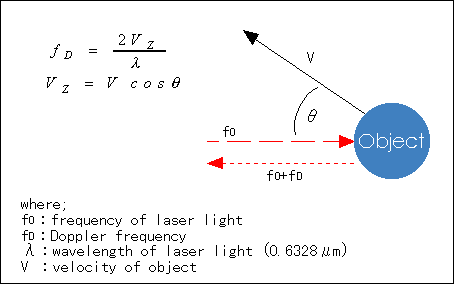As shown in the fugure above, the frequency of the reflected laser light is f0+fD. Since the wavelength I used by the laser Doppler vibrometer is extremely stable, the Doppler frequency fD and velocity V are proportional. Normally, the angle F is set at 0 (only the component of reflected light parallel to incident light--out-of-plane vibration*--is detected) and, therefore, the velocity of the object moving in the direction of the emitted laser light can be determined by measuring fD. The frequency of laser light is so high, however, that it is extremely difficult to measure directly. For this reason, fD is normally determined by allowing the emitted laser light (f0) to interfere with the reflected laser light (f0+fD).

Types of Laser Doppler Vibrometers

Laser Doppler vibrometers can be classified according to their structural design as shown below. The model numbers shown are the vibrometers produced by Ono Sokki for each respective class.

 1 Vibrometers for out-of-plane vibrtion / Reference-beam vibrometers LV-1710 / LV-1720A 2 Vibrometer for three-dimensional vibration LV-3300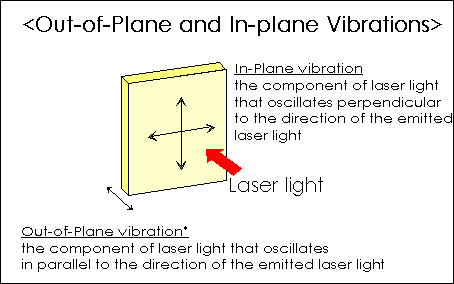The details of each type of vibrometer are given below.

Reference-beam vibrometers

These are the most basic laser Doppler vibrometers. The figure below illustrates how the LV-1710 and LV-1720A are configured.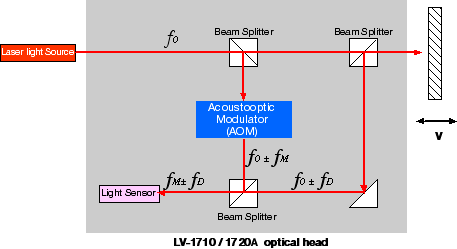Laser light released from the light source is split into two beams, one of which serves as an incident beam directed at the object under measurement, while the other serves as a reference beam fed back within the vibrometer. The beam reflected from the object experiences Doppler shift in proportion to the vibratory velocity of the object. This beam is then made to interfere with the reference beam, which is given a frequency shift beforehand by the acoustooptic modulator, so that a beat frequency can be obtained. From this beat frequency signal, only the Doppler-shift component is singled out at the detector circuit and sent to the FM demodulator to be output as a voltage signal that is proportional to the vibratory velocity.

Three-dimensional vibrometers

An object does not always vibrate in one direction only. It may in fact vibrate in a complex manner in three-dimensional directions. As products have become smaller in size and more precise, there has been an increasing need for measurement and analysis of three-dimensional, complex motion. The LV-3300 is a vibrometric system comprising three reference-beam laser Doppler vibrometer units. The system performs vector calculations when signals are received from these three vibrometers to simultaneously measure the X-, Y-, Z-axis vibratory velocity and the direction in which the object under measurement vibrates. The figure below illustrates how the optical heads of the LV-3300 system are configured and shows the equations for calculating the vectors.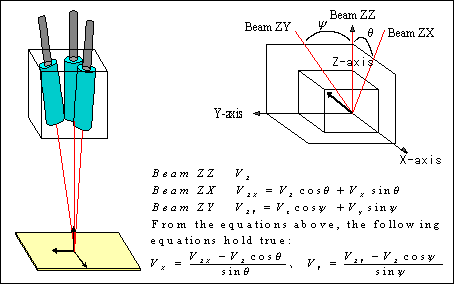One of the three optical heads is arranged so that the dirction of its incident light coincides with the direction (Z-axis) in which the object moves; while the other two are arranged so that the directions of their incident light(ZX and ZY) are specific angles away from the Z-axis. Signals from these angled optical heads represent the vibratory velocities and vibrations in the ZX and ZY directions. Consequently, beams reflected from all three optical heads contain signal components for the Z, ZX and ZY directions in which the object vibrates. Thus, we can measure the vibratory characteristics of the object for the X-,Y- and Z-axis directions simultaneously, by inputting these three signals into a vector calculator.

Advantages of laser Doppler vibrometers, along with precautions

· Non-contact measurement
· Wide dynamic range for measurement
· High spatial resolution

Precautions

· The sensor head must be placed directly opposite the object.
· The luminous energy of a reflected laser beam must be maintained at a given level.
· The vibrometer is susceptible to the effects of oil or water on an object.
· Extra care must be taken when measuring a rotating object since the vibrometer is affected by the noise caused by rough surfaces.

Supplementary Notes

1. Laser Doppler vibrometers do not affect the object under measurement due to their non-contact measurement method. The sensor head can be placed at a reasonable distance from the object (normally, 0.34 to 5m for the LV-1300, or more than 10m if combined with a commercially available lens). This feature enables us to measure even objects such as those that emit intense heat, without affecting the sensor head.

2. The LV-1300 has the following measurement ranges:
Velocity: 0.3Jm/s to 5m/s
Frequency:1Hz to 1.5MHz (can be increased up to 20MHz)
This means you can measure up to 0.01nm (0.00001Jm) in terms of displacement in the high-frequency domain.

3. The laser beam spot on an object is minutely small, measuring only several tens to several hundreds of microns wide (20Jm`400Jm for the LV-1300). Consequently, the vibration of even a microscopic object can be measured. If the sensor head is assembled in a dedicated microscope, the vibrometer can measure even smaller objects.

4. A feature of the LV-1100 is the extremely small sensor head, which is only about 10-mm wide and 40-mm long. The sensor head of the LV-1300 is also compact, measuring approximately 44mm~60mm~170mm for a type comprising an interferometer. The LV-1100 is therefore easy to handle and easily focused. Using a wide choice of optional mirrors, it is possible to measure vibration even at locations that are usually difficult to measure.

5. For vibrometers designed to measure out-of-plane vibration, the laser beam must be in line with the direction in which the object under measurement vibrates. If the laser beam is emitted at the object obliquely, the vibrometer detects the vector component given by the direction of the vibration and the laser beam as the amplitude of the vibration. Consequently, the vibrometer fails to obtain the correct vibration amplitude. In some cases, it may even fail to detect any amplitude if the angle between the direction in which the laser beam runs and the direction in which the object vibrates is too large.

6. A laser Doppler vibrometr uses the same optical channel for both the projection section and the receiving section. If the luminous energy of the laser light, which is reflected from the object and returns to the light sensor, is below a given level, the signal-to-noise ratio deteriorates. This deterioration sometimes results in a a failure to perform measurement. For this reason, the laser Doppler vibrometer is best used for objects that reflect beams in completely opposite directions. This vibrometer can also measure objects that scatter beams, if the luminous energy of the reflected laser light, which returns to the object lens in the sensor head, is above the specified level. More specifically, even objects such as black rubber that reflect little luminous energy can be measured using LV-1100/1500 vibrometers if the returned energy is at least 4% of the original, or using the LV-1300 vibrometer if the sensor head is close to the object. If the lumious energy is likely to be inadequate, reflexive materials such as a reflection sheet or reflective paint must be attached to or coated on the object.

7. Vibrometric measurement is not possible if oil or water is flowing on the surfaces of the object being measured. Measurement error will result if the object is wet with oil or water; whenever possible, such foreign matter must be removed. These problems are due to the unwanted reflection of laesr beams at the surface of an oil or water film. Such reflection may not only prevent the vibrometer from precisely measuring the vibration amplitude of the object, but may also lead to an inablity to measure due to random reflection.

8. Care must be taken when measuring such objects as a rotating body that has a significant vibratory or motional component that is perpendicular to the direction of a laser beam. Depending on the roughness and shape of the object surface, a momentary absence of reflected laser light may occur. This can result in the appearance of spike noise in the output signal, leading to measurement errors.

(6) Torsion Vibrometers

Torsional vibration takes place as an object rotates. This type of vibration can occur for different reasons. In a rotational system where the drive unit and load section are directly coupled, such vibration is caused by a change in the revolution of the rotating part. In a multi-shaft system where gears, belts and chains are interconnected, such vibration is caused by a transfer error resulting from poor machining accuracy or a deformity in the power transmissions, or from a change in the revolution of the rotating parts. Normally, revolution changes, torsional vibration and transfer errors are intermingled in a complex manner. A variety of adverse effects develop in systems which experience these, such as:

1.Increase in vibration and/or noise
2.Deterioration in the positioning accuracy
3.Deterioration in the feed accuracy
4.Breakdown due to fatigue

Therefore, in a system comprising rotating parts and power transmissions, it is important to measure these three parameters--revolution change, torsional vibration and transmission error--in order to analyze the cause-and-effect relationship.

Changes in Revolution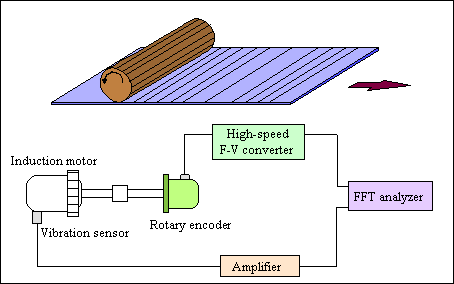A change in revolution can be due to either an increase or decrease in the rotational angular velocity against the average angular velocity at a particular point on a rotating shaft. In this measurement, a high-resolution rotary encoder is used. The output signal from the encoder is converted from frequency to voltage (F-V) at high speeds, pulse by pulse, to deterimine the change in each revolution.

Torsional Vibration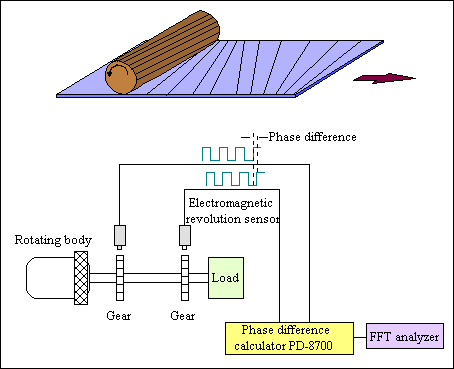Torsional vibration represents changes in the relative angular displacement between two points on a rotating shaft. Normally, a pair of electromagnetic revolution sensors are used for this measurement. The vibrometer measures the torsion that develops across the driving and load sides of the shaft as the phase difference. The phase difference obtrained is F-V converted at high speeds and processed using frequency calculations to determine the torsional vibration.

Transission Errors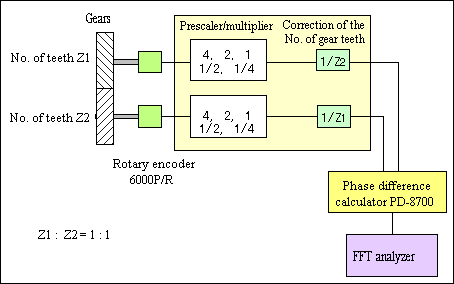A transmission error is the lead or lag in the rotational angle at the front and back locations of a power transmission unit in a multi-shaft rotary machine. This is measured from the phase difference between the front and back locations of a power transmission, similar to the detection of torsional vibration noted earlier. The per-pitch transmission error is determined from the phase difference, and then processed by frequency calculations to determine the transmission error.

PD-8700 Torsional Angle Converter (Discontinue Product)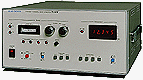Designed to execute 16-bit, precision digital computation and pulse-mode computation over four periods, this converter makes it possible to measure relative angular displacements at a resolution more than 16 times higher than conventional converters, for applications where phase differences between pulses are measured to determine torsional vibration, transfer errors in gears, and other variables.

The following are cross-conversion equations used to find acceleration, velocity and displacement.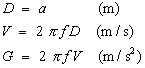D Displacement V Velocity G Acceleration f frequency

If frequency f is known and one of the variables D, V and G is measured, the other two physical quantities can be determined by calculation.

In the next issue of the ONO SOKKI TECHNICAL REPORT, we will discuss"sound and sensors".